# Keyword Spotting in Noise Using MFCC and LSTM Networks

This example shows how to identify a keyword in noisy speech using a deep learning network. In particular, the example uses a Bidirectional Long Short-Term Memory (BiLSTM) network and mel-frequency cepstral coefficients (MFCC).

### Introduction

Keyword spotting (KWS) is an essential component of voice-assist technologies, where the user speaks a predefined keyword to wake-up a system before speaking a complete command or query to the device.

This example trains a KWS deep network with feature sequences of mel-frequency cepstral coefficients (MFCC). The example also demonstrates how network accuracy in a noisy environment can be improved using data augmentation.

This example uses long short-term memory (LSTM) networks, which are a type of recurrent neural network (RNN) well-suited to study sequence and time-series data. An LSTM network can learn long-term dependencies between time steps of a sequence. An LSTM layer (`lstmLayer`) can look at the time sequence in the forward direction, while a bidirectional LSTM layer (`bilstmLayer`) can look at the time sequence in both forward and backward directions. This example uses a bidirectional LSTM layer.

The example uses the google Speech Commands Dataset to train the deep learning model. To run the example, you must first download the data set. If you do not want to download the data set or train the network, then you can load a pretrained network by opening this example in MATLAB® and typing `load("KWSNet.mat")` at the command line.

### Example Summary

The example goes through the following steps:

1. Inspect a "gold standard" keyword spotting baseline on a validation signal.

2. Create training utterances from a noise-free dataset.

3. Train a keyword spotting LSTM network using MFCC sequences extracted from those utterances.

4. Check the network accuracy by comparing the validation baseline to the output of the network when applied to the validation signal.

5. Check the network accuracy for a validation signal corrupted by noise.

6. Augment the training dataset by injecting noise to the speech data using `audioDataAugmenter`.

7. Retrain the network with the augmented dataset.

8. Verify that the retrained network now yields higher accuracy when applied to the noisy validation signal.

### Inspect the Validation Signal

In this example, the keyword to spot is YES.

You use a sample speech signal to validate the KWS network. The validation signal consists 34 seconds of speech with the keyword YES appearing intermittently.

`[audioIn,fs] = audioread('KeywordSpeech-16-16-mono-34secs.flac');`

Listen to the signal.

`sound(audioIn,fs)`

Visualize the signal.

```t = (1/fs) * (0:length(audioIn)-1); plot(t,audioIn); grid on xlabel('Time (s)') title('Validation Speech Signal')```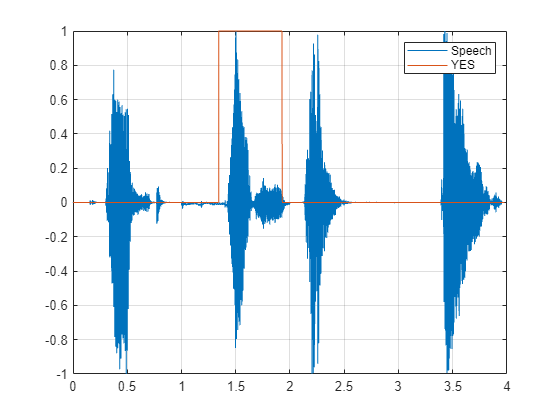### Inspect the KWS Baseline

Load the KWS baseline. This baseline was obtained using `speech2text`: Create Keyword Spotting Mask Using Audio Labeler.

`load('KWSBaseline.mat','KWSBaseline')`

The baseline is a logical vector of the same length as the validation audio signal. Segments in `audioIn` where the keyword is uttered are set to one in `KWSBaseline`.

Visualize the speech signal along with the KWS baseline.

```fig = figure; plot(t,[audioIn,KWSBaseline']) grid on xlabel('Time (s)') legend('Speech','KWS Baseline','Location','southeast') l = findall(fig,'type','line'); l(1).LineWidth = 2; title("Validation Signal")```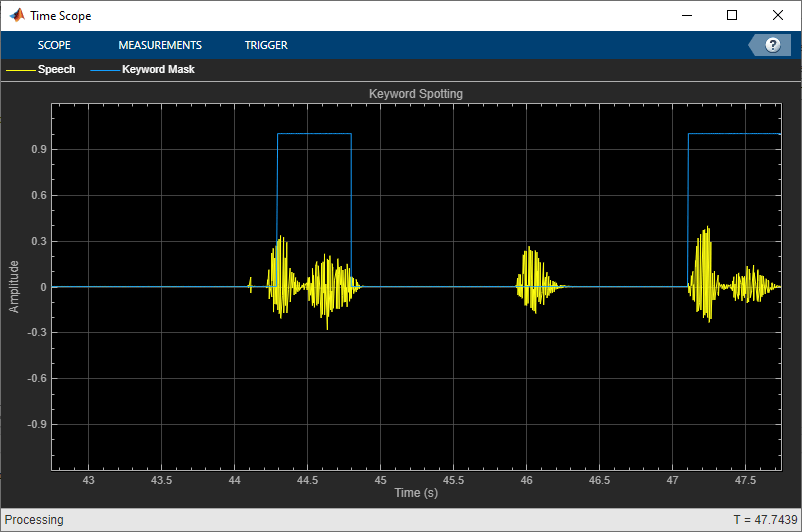Listen to the speech segments identified as keywords.

`sound(audioIn(KWSBaseline),fs)`

The objective of the network that you train is to output a KWS mask of zeros and ones like this baseline.

### Load Speech Commands Data Set

```url = 'https://storage.googleapis.com/download.tensorflow.org/data/speech_commands_v0.01.tar.gz'; downloadFolder = tempdir; datasetFolder = fullfile(downloadFolder,'google_speech'); if ~exist(datasetFolder,'dir') disp('Downloading Google speech commands data set (1.5 GB)...') untar(url,datasetFolder) end```

Create an `audioDatastore` that points to the data set.

`ads = audioDatastore(datasetFolder,'LabelSource','foldername','Includesubfolders',true);`

The dataset contains background noise files that are not used in this example. Use `subset` to create a new datastore that does not have the background noise files.

```isBackNoise = ismember(ads.Labels,"_background_noise_"); ads = subset(ads,~isBackNoise);```

The dataset has approximately 65,000 one-second long utterances of 30 short words (including the keyword YES). Get a breakdown of the word distribution in the datastore.

`countEachLabel(ads)`
```ans=30×2 table Label Count ______ _____ bed 1713 bird 1731 cat 1733 dog 1746 down 2359 eight 2352 five 2357 four 2372 go 2372 happy 1742 house 1750 left 2353 marvin 1746 nine 2364 no 2375 off 2357 ⋮ ```

Split `ads` into two datastores: The first datastore contains files corresponding to the keyword. The second datastore contains all the other words.

```keyword = 'yes'; isKeyword = ismember(ads.Labels,keyword); ads_keyword = subset(ads,isKeyword); ads_other = subset(ads,~isKeyword);```

To train the network with the entire dataset and achieve the highest possible accuracy, set `reduceDataset` to `false`. To run this example quickly, set `reduceDataset` to `true`.

```reduceDataset = false; if reduceDataset % Reduce the dataset by a factor of 20 ads_keyword = splitEachLabel(ads_keyword,round(numel(ads_keyword.Files) / 20)); numUniqueLabels = numel(unique(ads_other.Labels)); ads_other = splitEachLabel(ads_other,round(numel(ads_other.Files) / numUniqueLabels / 20)); end```

Get a breakdown of the word distribution in each datastore. Shuffle the `ads_other` datastore so that consecutive reads return different words.

`countEachLabel(ads_keyword)`
```ans=1×2 table Label Count _____ _____ yes 2377 ```
`countEachLabel(ads_other)`
```ans=29×2 table Label Count ______ _____ bed 1713 bird 1731 cat 1733 dog 1746 down 2359 eight 2352 five 2357 four 2372 go 2372 happy 1742 house 1750 left 2353 marvin 1746 nine 2364 no 2375 off 2357 ⋮ ```
`ads_other = shuffle(ads_other);`

### Create Training Sentences and Labels

The training datastores contain one-second speech signals where one word is uttered. You will create more complex training speech utterances that contain a mixture of the keyword along with other words.

Here is an example of a constructed utterance. Read one keyword from the keyword datastore and normalize it to have a maximum value of one.

```yes = read(ads_keyword); yes = yes / max(abs(yes));```

The signal has non-speech portions (silence, background noise, etc.) that do not contain useful speech information. This example removes silence using `detectSpeech`.

Get the start and end indices of the useful portion of the signal.

`speechIndices = detectSpeech(yes,fs);`

Randomly select the number of words to use in the synthesized training sentence. Use a maximum of 10 words.

`numWords = randi([0 10]);`

Randomly pick the location at which the keyword occurs.

`keywordLocation = randi([1 numWords+1]);`

Read the desired number of non-keyword utterances, and construct the training sentence and mask.

```sentence = []; mask = []; for index = 1:numWords+1 if index == keywordLocation sentence = [sentence;yes]; %#ok newMask = zeros(size(yes)); newMask(speechIndices(1,1):speechIndices(1,2)) = 1; mask = [mask;newMask]; %#ok else other = read(ads_other); other = other ./ max(abs(other)); sentence = [sentence;other]; %#ok mask = [mask;zeros(size(other))]; %#ok end end```

Plot the training sentence along with the mask.

```figure t = (1/fs) * (0:length(sentence)-1); fig = figure; plot(t,[sentence,mask]) grid on xlabel('Time (s)') legend('Training Signal','Mask','Location','southeast') l = findall(fig,'type','line'); l(1).LineWidth = 2; title("Example Utterance")```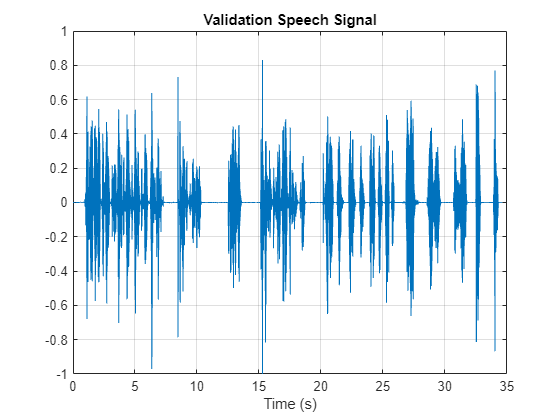Listen to the training sentence.

`sound(sentence,fs)`

### Extract Features

This example trains a deep learning network using 42 MFCC coefficients (14 MFCC, 14 delta and 14 delta-delta coefficients).

Define parameters required for MFCC extraction.

```WindowLength = 512; OverlapLength = 384;```

Extract the MFCC features.

`[coeffs,delta,deltaDelta] = mfcc(sentence,fs,'WindowLength',WindowLength,'OverlapLength',OverlapLength);`

Concatenate the coefficients into one feature matrix.

```featureMatrix = [coeffs, delta, deltaDelta]; size(featureMatrix)```
```ans = 1×2 1085 42 ```

Note that you compute MFCC by sliding a window through the input, so the feature matrix is shorter than the input speech signal. Each row in `featureMatrix` corresponds to 128 samples from the speech signal (`WindowLength`-`OverlapLength`).

Compute a mask of the same length as `featureMatrix`.

```HopLength = WindowLength - OverlapLength; range = HopLength * (1:size(coeffs,1)) + HopLength; featureMask = zeros(size(range)); for index = 1:numel(range) featureMask(index) = mode(mask( (index-1)*HopLength+1:(index-1)*HopLength+WindowLength )); end```

### Extract Features from Training Dataset

Sentence synthesis and feature extraction for the whole training dataset can be quite time-consuming. To speed up processing, if you have Parallel Computing Toolbox™, partition the training datastore, and process each partition on a separate worker.

Select a number of datastore partitions.

`numPartitions = 6;`

Initialize cell arrays for the feature matrices and masks.

```TrainingFeatures = {}; TrainingMasks = {};```

Perform sentence synthesis, feature extraction, and mask creation using `parfor`.

```tic parfor ii = 1:numPartitions subads_keyword = partition(ads_keyword,numPartitions,ii); subads_other = partition(ads_other,numPartitions,ii); count = 1; localFeatures = cell(length(subads_keyword.Files),1); localMasks = cell(length(subads_keyword.Files),1); while hasdata(subads_keyword) % Create a training sentence [sentence,mask] = HelperSynthesizeSentence(subads_keyword,subads_other,fs,WindowLength); % Compute mfcc features [coeffs,delta,deltaDelta] = mfcc(sentence,fs,'WindowLength',WindowLength,'OverlapLength',OverlapLength); featureMatrix = [coeffs, delta, deltaDelta]; featureMatrix(~isfinite(featureMatrix)) = 0; % Create mask hopLength = WindowLength - OverlapLength; range = (hopLength) * (1:size(coeffs,1)) + hopLength; featureMask = zeros(size(range)); for index = 1:numel(range) featureMask(index) = mode(mask( (index-1)*hopLength+1:(index-1)*hopLength+WindowLength )); end localFeatures{count} = featureMatrix; catVect = categorical(featureMask); catVect = addcats(catVect,{'1'}); localMasks{count} = catVect; count = count + 1; end TrainingFeatures = [TrainingFeatures;localFeatures]; TrainingMasks = [TrainingMasks;localMasks]; end```
```Starting parallel pool (parpool) using the 'local' profile ... Connected to the parallel pool (number of workers: 6). ```
`fprintf('Training feature extraction took %f seconds.\n',toc)`
```Training feature extraction took 101.747986 seconds. ```

It is good practice to normalize all features to have zero mean and unity standard deviation. Compute the mean and standard deviation for each coefficient and use them to normalize the data.

```sampleFeature = TrainingFeatures{1}; numFeatures = size(sampleFeature,2); featuresMatrix = cat(1,TrainingFeatures{:}); M = mean(featuresMatrix); S = std(featuresMatrix); for index = 1:length(TrainingFeatures) f = TrainingFeatures{index}; f = (f - M) ./ S; TrainingFeatures{index} = f.'; %#ok end```

### Extract Validation Features

Extract MFCC features from the validation signal.

```[coeffs,delta,deltaDelta] = mfcc(audioIn,fs,'WindowLength',WindowLength,'OverlapLength',OverlapLength); featureMatrix = [coeffs, delta, deltaDelta]; featureMatrix(~isfinite(featureMatrix)) = 0;```

Normalize the validation features.

```FeaturesValidationClean = (featureMatrix - M)./S; range = HopLength * (1:size(FeaturesValidationClean,1)) + HopLength;```

```featureMask = zeros(size(range)); for index = 1:numel(range) featureMask(index) = mode(KWSBaseline( (index-1)*HopLength+1:(index-1)*HopLength+WindowLength )); end BaselineV = categorical(featureMask);```

### Define the LSTM Network Architecture

LSTM networks can learn long-term dependencies between time steps of sequence data. This example uses the bidirectional LSTM layer `bilstmLayer` to look at the sequence in both forward and backward directions.

Specify the input size to be sequences of size `numFeatures`. Specify two hidden bidirectional LSTM layers with an output size of 150 and output a sequence. This command instructs the bidirectional LSTM layer to map the input time series into 150 features that are passed to the next layer. Specify two classes by including a fully connected layer of size 2, followed by a softmax layer and a classification layer.

```layers = [ ... sequenceInputLayer(numFeatures) bilstmLayer(150,"OutputMode","sequence") bilstmLayer(150,"OutputMode","sequence") fullyConnectedLayer(2) softmaxLayer classificationLayer ];```

### Define Training Options

Specify the training options for the classifier. Set `MaxEpochs` to 10 so that the network makes 10 passes through the training data. Set `MiniBatchSize` to `64` so that the network looks at 64 training signals at a time. Set `Plots` to `"training-progress"` to generate plots that show the training progress as the number of iterations increases. Set `Verbose` to `false` to disable printing the table output that corresponds to the data shown in the plot. Set `Shuffle` to `"every-epoch"` to shuffle the training sequence at the beginning of each epoch. Set `LearnRateSchedule` to `"piecewise"` to decrease the learning rate by a specified factor (0.1) every time a certain number of epochs (5) has passed. Set `ValidationData` to the validation predictors and targets.

This example uses the adaptive moment estimation (ADAM) solver. ADAM performs better with recurrent neural networks (RNNs) like LSTMs than the default stochastic gradient descent with momentum (SGDM) solver.

```maxEpochs = 10; miniBatchSize = 64; options = trainingOptions("adam", ... "InitialLearnRate",1e-4, ... "MaxEpochs",maxEpochs, ... "MiniBatchSize",miniBatchSize, ... "Shuffle","every-epoch", ... "Verbose",false, ... "ValidationFrequency",floor(numel(TrainingFeatures)/miniBatchSize), ... "ValidationData",{FeaturesValidationClean.',BaselineV}, ... "Plots","training-progress", ... "LearnRateSchedule","piecewise", ... "LearnRateDropFactor",0.1, ... "LearnRateDropPeriod",5);```

### Train the LSTM Network

Train the LSTM network with the specified training options and layer architecture using `trainNetwork`. Because the training set is large, the training process can take several minutes.

```if ~reduceDataset [keywordNetNoAugmentation,netInfo] = trainNetwork(TrainingFeatures,TrainingMasks,layers,options); fprintf("Validation accuracy: %f percent.\n",netInfo.FinalValidationAccuracy); else load('keywordNetNoAugmentation.mat','keywordNetNoAugmentation','M','S');%#ok end```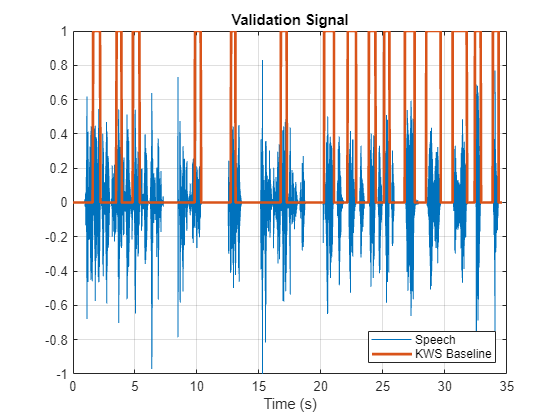```Validation accuracy: 95.255728 percent. ```

### Check Network Accuracy for a Noise-Free Validation Signal

Estimate the KWS mask for the validation signal using the trained network.

`v = classify(keywordNetNoAugmentation,FeaturesValidationClean.');`

Calculate and plot the validation confusion matrix from the vectors of actual and estimated labels.

```figure cm = confusionchart(BaselineV,v,"title","Validation Accuracy"); cm.ColumnSummary = "column-normalized"; cm.RowSummary = "row-normalized";```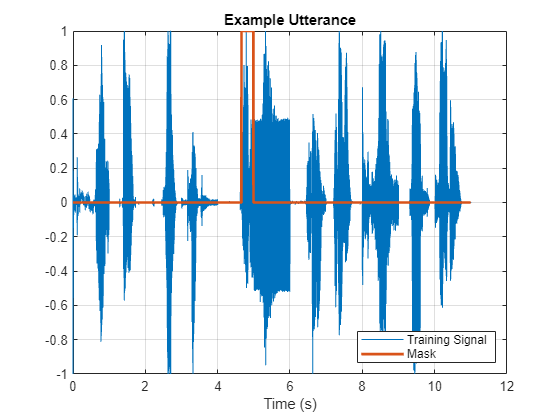Convert the network output from categorical to double.

```v = double(v) - 1; v = repmat(v,HopLength,1); v = v(:);```

Listen to the keyword areas identified by the network.

`sound(audioIn(logical(v)),fs)`

Visualize the estimated and expected KWS masks.

```baseline = double(BaselineV) - 1; baseline = repmat(baseline,HopLength,1); baseline = baseline(:); t = (1/fs) * (0:length(v)-1); fig = figure; plot(t,[audioIn(1:length(v)),v,0.8*baseline]) grid on xlabel('Time (s)') legend('Training Signal','Network Mask','Baseline Mask','Location','southeast') l = findall(fig,'type','line'); l(1).LineWidth = 2; l(2).LineWidth = 2; title('Results for Noise-Free Speech')```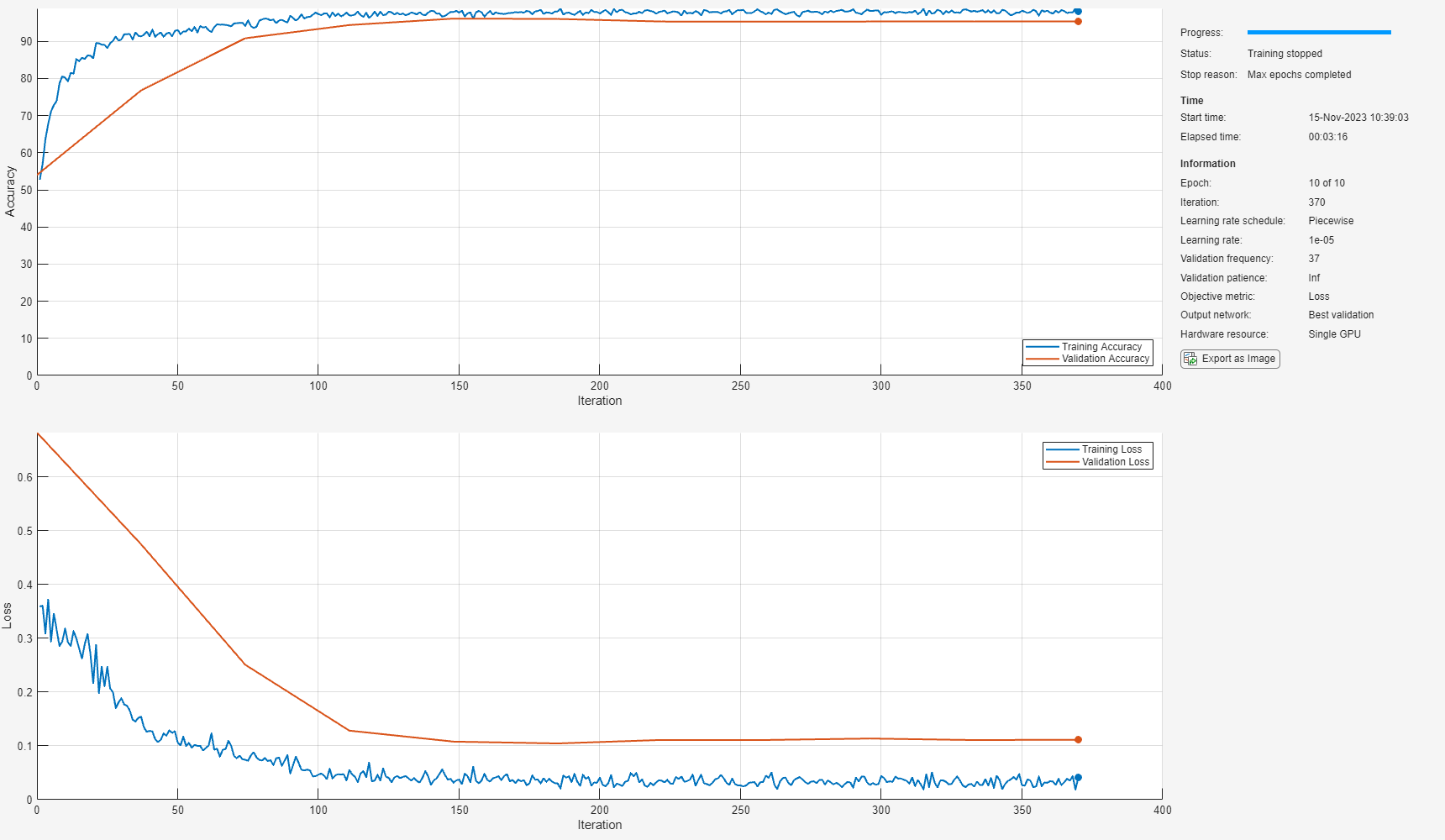### Check Network Accuracy for a Noisy Validation Signal

You will now check the network accuracy for a noisy speech signal. The noisy signal was obtained by corrupting the clean validation signal by additive white Gaussian noise.

```[audioInNoisy,fs] = audioread('NoisyKeywordSpeech-16-16-mono-34secs.flac'); sound(audioInNoisy,fs)```

Visualize the signal.

```figure t = (1/fs) * (0:length(audioInNoisy)-1); plot(t,audioInNoisy) grid on xlabel('Time (s)') title('Noisy Validation Speech Signal')```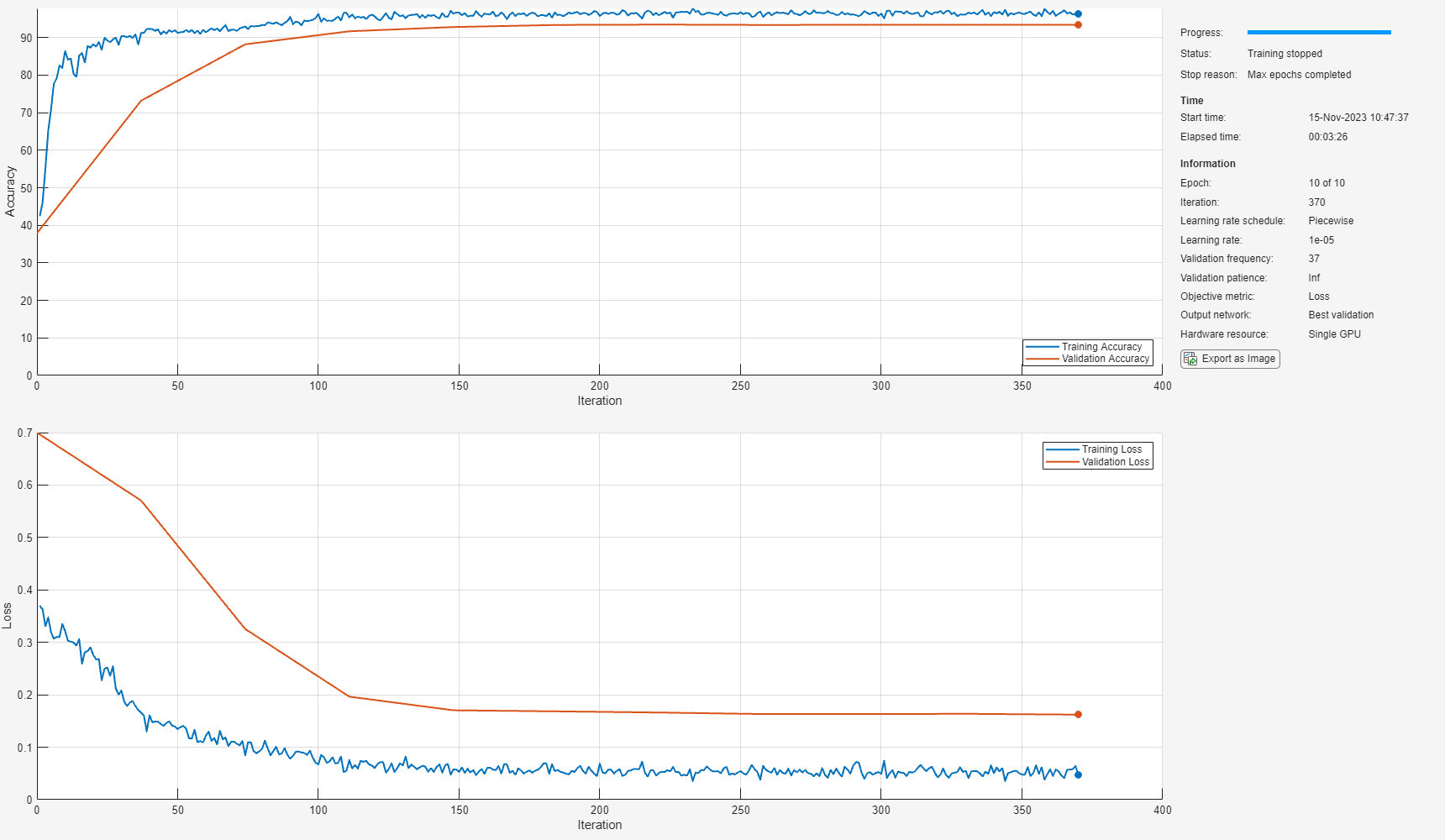Extract the feature matrix from the noisy signal.

```[coeffs,delta,deltaDelta] = mfcc(audioInNoisy,fs,'WindowLength',WindowLength,'OverlapLength',OverlapLength); featureMatrixV = [coeffs, delta, deltaDelta]; featureMatrixV(~isfinite(featureMatrixV)) = 0; FeaturesValidationNoisy = (featureMatrixV - M)./S;```

Pass the feature matrix to the network.

`v = classify(keywordNetNoAugmentation,FeaturesValidationNoisy.');`

Compare the network output to the baseline. Note that the accuracy is lower than the one you got for a clean signal.

```figure cm = confusionchart(BaselineV,v,"title","Validation Accuracy - Noisy Speech"); cm.ColumnSummary = "column-normalized"; cm.RowSummary = "row-normalized";```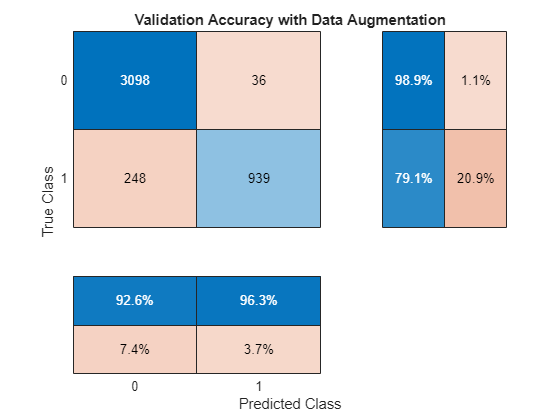Convert the network output from categorical to double.

``` v = double(v) - 1; v = repmat(v,HopLength,1); v = v(:);```

Listen to the keyword areas identified by the network.

`sound(audioIn(logical(v)),fs)`

Visualize the estimated and baseline masks.

```t = (1/fs)*(0:length(v)-1); fig = figure; plot(t,[audioInNoisy(1:length(v)),v,0.8*baseline]) grid on xlabel('Time (s)') legend('Training Signal','Network Mask','Baseline Mask','Location','southeast') l = findall(fig,'type','line'); l(1).LineWidth = 2; l(2).LineWidth = 2; title('Results for Noisy Speech - No Data Augmentation')```### Perform Data Augmentation

The trained network did not perform well on a noisy signal because the trained dataset contained only noise-free sentences. You will rectify this by augmenting your dataset to include noisy sentences.

Use `audioDataAugmenter` to augment your dataset.

```ada = audioDataAugmenter('TimeStretchProbability',0, ... 'PitchShiftProbability',0, ... 'VolumeControlProbability',0, ... 'TimeShiftProbability',0, ... 'SNRRange',[-1, 1], ... 'AddNoiseProbability',0.85);```

With these settings, the `audioDataAugmenter` object corrupts an input audio signal with white Gaussian noise with a probability of 85%. The SNR is randomly selected from the range [-1 1] (in dB). There is a 15% probability that the augmenter does not modify your input signal.

As an example, pass an audio signal to the augmenter.

```reset(ads_keyword) x = read(ads_keyword); data = augment(ada,x,fs)```
```data=1×2 table Audio AugmentationInfo ________________ ________________ {16000×1 double} [1×1 struct] ```

Inspect the `AugmentationInfo` variable in `data` to verify how the signal was modified.

`data.AugmentationInfo`
```ans = struct with fields: SNR: -0.1243 ```

Reset the datastores.

```reset(ads_keyword) reset(ads_other)```

Initialize the feature and mask cells.

```TrainingFeatures = {}; TrainingMasks = {};```

Perform feature extraction again. Each signal is corrupted by noise with a probability of 85%, so your augmented dataset has approximately 85% noisy data and 15% noise-free data.

```tic parfor ii = 1:numPartitions subads_keyword = partition(ads_keyword,numPartitions,ii); subads_other = partition(ads_other,numPartitions,ii); count = 1; localFeatures = cell(length(subads_keyword.Files),1); localMasks = cell(length(subads_keyword.Files),1); while hasdata(subads_keyword) [sentence,mask] = HelperSynthesizeSentence(subads_keyword,subads_other,fs,WindowLength); % Corrupt with noise augmentedData = augment(ada,sentence,fs); sentence = augmentedData.Audio{1}; % Compute mfcc features [coeffs,delta,deltaDelta] = mfcc(sentence,fs,'WindowLength',WindowLength,'OverlapLength',OverlapLength); featureMatrix = [coeffs delta deltaDelta]; featureMatrix(~isfinite(featureMatrix)) = 0; hopLength = WindowLength - OverlapLength; range = hopLength * (1:size(coeffs,1)) + hopLength; featureMask = zeros(size(range)); for index = 1:numel(range) featureMask(index) = mode(mask( (index-1)*hopLength+1:(index-1)*hopLength+WindowLength )); end localFeatures{count} = featureMatrix; catVect = categorical(featureMask); catVect = addcats(catVect,{'1'}); localMasks{count} = catVect; count = count + 1; end TrainingFeatures = [TrainingFeatures;localFeatures]; TrainingMasks = [TrainingMasks;localMasks]; end fprintf('Training feature extraction took %f seconds.\n',toc)```
```Training feature extraction took 41.517132 seconds. ```

Compute the mean and standard deviation for each coefficient; use them to normalize the data.

```sampleFeature = TrainingFeatures{1}; numFeatures = size(sampleFeature,2); featuresMatrix = cat(1,TrainingFeatures{:}); M = mean(featuresMatrix); S = std(featuresMatrix); for index = 1:length(TrainingFeatures) f = TrainingFeatures{index}; f = (f - M) ./ S; TrainingFeatures{index} = f.'; %#ok end```

Normalize the validation features with the new mean and standard deviation values.

`FeaturesValidationNoisy = (featureMatrixV - M)./S;`

### Retrain Network with Augmented Dataset

Recreate the training options. Use the noisy baseline features and mask for validation.

```options = trainingOptions("adam", ... "InitialLearnRate",1e-4, ... "MaxEpochs",maxEpochs, ... "MiniBatchSize",miniBatchSize, ... "Shuffle","every-epoch", ... "Verbose",false, ... "ValidationFrequency",floor(numel(TrainingFeatures)/miniBatchSize), ... "ValidationData",{FeaturesValidationNoisy.',BaselineV}, ... "Plots","training-progress", ... "LearnRateSchedule","piecewise", ... "LearnRateDropFactor",0.1, ... "LearnRateDropPeriod",5);```

Train the network.

```if ~reduceDataset [KWSNet,netInfo] = trainNetwork(TrainingFeatures,TrainingMasks,layers,options); else load('KWSNet.mat','KWSNet');%#ok end```Verify the network accuracy on the validation signal.

`v = classify(KWSNet,FeaturesValidationNoisy.');`

Compare the estimated and expected KWS masks.

```figure cm = confusionchart(BaselineV,v,"title","Validation Accuracy with Data Augmentation"); cm.ColumnSummary = "column-normalized"; cm.RowSummary = "row-normalized";```Listen to the identified keyword regions.

```v = double(v) - 1; v = repmat(v,HopLength,1); v = v(:); sound(audioIn(logical(v)),fs)```

Visualize the estimated and expected masks.

```fig = figure; plot(t,[audioInNoisy(1:length(v)),v,0.8*baseline]) grid on xlabel('Time (s)') legend('Training Signal','Network Mask','Baseline Mask','Location','southeast') l = findall(fig,'type','line'); l(1).LineWidth = 2; l(2).LineWidth = 2; title('Results for Noisy Speech - With Data Augmentation')```### References

```function [sentence,mask] = HelperSynthesizeSentence(ads_keyword,ads_other,fs,minlength) % Read one keyword keyword = read(ads_keyword); keyword = keyword ./ max(abs(keyword)); % Identify region of interest speechIndices = detectSpeech(keyword,fs); if isempty(speechIndices) || diff(speechIndices(1,:)) <= minlength speechIndices = [1,length(keyword)]; end keyword = keyword(speechIndices(1,1):speechIndices(1,2)); % Pick a random number of other words (between 0 and 10) numWords = randi([0 10]); % Pick where to insert keyword loc = randi([1 numWords+1]); sentence = []; mask = []; for index = 1:numWords+1 if index==loc sentence = [sentence;keyword]; newMask = ones(size(keyword)); mask = [mask ;newMask]; else other = read(ads_other); other = other ./ max(abs(other)); sentence = [sentence;other]; mask = [mask;zeros(size(other))]; end end end```